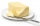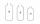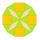# Volume + divisibility - math problems

#### Number of problems found: 8

• Eights of butterHow many eights of butter (1/8 of kg = 125 g) can be stored in a box with dimensions of 4 dm, 2 dm, 1.8 dm, if the eighth of butter has dimensions of 8 cm, 5 cm, 3 cm?
• Integer cubeThe length of the cube edge is an integer. Its volume is in cm3 a five-digit number divisible by 1331. What is the length of the edge of this cube.
• An exampleAn example is playfully for grade 6 from Math and I don't know how to explain it to my daughter when I don't want to use the calculator to calculate the cube root. Thus: A cuboid was made from a block of 16x18x48 mm of modeline. What will be the edge of t
• Bricks pyramidHow many 50cm x 32cm x 30cm brick needed to built a 272m x 272m x 278m pyramid?
• Cuboid wallsCalculate the cuboid volume if its different walls have an area of 195cm², 135cm², and 117cm².
• Cube-shaped boxDesign the size of the smallest possible cube-shaped box where three types of 3cm, 5cm, 6cm small cubes could be stacked to make full use of the box space (each type of cube separately). Can you find out how many smallest cubes are in the box?
• FluidWe have vessels containing 7 liters, 5 liters, and 2 liters. The largest container is filled with fluid, the others empty. Can you only by pouring get 5 liters and two 1 liter of fluid? How much pouring is needed?
• CuboidThe volume of the cuboid is 245 cm3. Each cuboid edge length can be expressed by an integer greater than 1 cm. What is the surface area of the cuboid?

We apologize, but in this category are not a lot of examples.
Do you have an exciting math question or word problem that you can't solve? Ask a question or post a math problem, and we can try to solve it.

We will send a solution to your e-mail address. Solved examples are also published here. Please enter the e-mail correctly and check whether you don't have a full mailbox.

Tip: Our volume units converter will help you with the conversion of volume units. Volume - math problems. Divisibility - math problems.